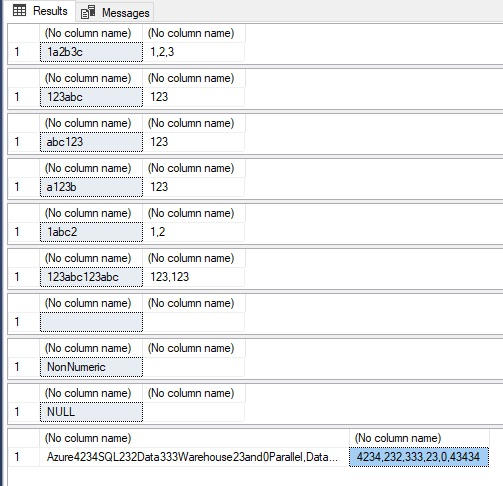# Extract Numbers From String In SQL Server

I was looking for options to retrieve numbers from an alpha numeric string. I could find code to remove alpha characters from the string leaving the numeric characters forming one large number. However, my requirement is to extract the number sets and separate them with commas. i.e. if there is an alpha numeric string abc123def456ghi789 the numbers should be segregated as 123,456,789. So, I wrote a small user defined function to extract the numbers in sets.

## Function To Extract Numbers From String

```CREATE FUNCTION [dbo].[mtb_GetNumbers]
(
@stInput VARCHAR(max)
)
RETURNS VARCHAR(max)
AS
BEGIN

SET @stInput = REPLACE(@stInput,',','')

DECLARE @intAlpha INT
DECLARE @intNumber INT

SET @intAlpha = PATINDEX('%[^0-9,]%', @stInput)
SET @intNumber = PATINDEX('%[0-9,]%', @stInput)

IF @stInput IS NULL OR @intNumber = 0
RETURN '';

WHILE @intAlpha > 0
BEGIN
IF (@intAlpha > @intNumber)
BEGIN
SET @intNumber = PATINDEX('%[0-9,]%', SUBSTRING(@stInput, @intAlpha, LEN(@stInput)) )
SELECT @intNumber = CASE WHEN @intNumber = 0 THEN LEN(@stInput) ELSE @intNumber END
END

SET @stInput = STUFF(@stInput, @intAlpha, @intNumber - 1,',' );

SET @intAlpha = PATINDEX('%[^0-9,]%', @stInput )
SET @intNumber = PATINDEX('%[0-9,]%', SUBSTRING(@stInput, @intAlpha, LEN(@stInput)) )
SELECT @intNumber = CASE WHEN @intNumber = 0 THEN LEN(@stInput) ELSE @intNumber END
END

IF (RIGHT(@stInput, 1) = ',')
SET @stInput = LEFT(@stInput, LEN(@stInput) - 1)

IF (LEFT(@stInput, 1) = ',')
SET @stInput = RIGHT(@stInput, LEN(@stInput) - 1)

RETURN ISNULL(@stInput,0)
END
GO
```

## Testing The Function

```/* Testing the function with alpha numeric strings */
SELECT '1a2b3c',  dbo.mtb_GetNumbers('1a2b3c');
SELECT '123abc',  dbo.mtb_GetNumbers('123abc');
SELECT 'abc123',  dbo.mtb_GetNumbers('abc123');
SELECT 'a123b',  dbo.mtb_GetNumbers('a123b');
SELECT '1abc2',  dbo.mtb_GetNumbers('1abc2');
SELECT '123abc123abc',  dbo.mtb_GetNumbers('123abc123abc');
SELECT '',  dbo.mtb_GetNumbers('');
SELECT 'NonNumeric', dbo.mtb_GetNumbers('NonNumeric');
SELECT 'NULL', dbo.mtb_GetNumbers(NULL);
SELECT 'Azure4234SQL232Data333Warehouse23and0Parallel,Data43434Warehouse',  dbo.mtb_GetNumbers('Azure4234SQL232Data333Warehouse23and0Parallel,Data43434Warehouse');
GO
```

### Result

Now we get the numbers as a string segregated with commas. If you want to split this delimited numbers, use STRING_SPLIT() in SQL Server 2016  or other options in older versions as explained here.

### 2 thoughts on “Extract Numbers From String In SQL Server”

1.This is excellent, But something seems to be wrong with the syntax when I run it?

i get this error

Msg 102, Level 15, State 1, Procedure fn_GetNumbers, Line 19 [Batch Start Line 0]
Incorrect syntax near ‘;’.
Msg 102, Level 15, State 1, Procedure fn_GetNumbers, Line 21 [Batch Start Line 0]
Incorrect syntax near ‘;’.

•